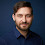### Python tidbits: inverting the nesting of a nested list.

More than once I came across the problem of rearranging a nested list. I had a nested list of the form
```
X = [['a', 'b', 'c'], ['d', 'e', 'f']]

```
And I want
```
Y = [['a', 'd'], ['b', 'e'], ['c', 'f']]

```
without having to resort to an ugly list comprehension over 3 lines. A friend told me to use
```
Y = zip(*X)

```
So easy! Kind of obvious but I didn't find it on the web. So I thought I'd write it down. Enjoy!

1.>>> X = [['a', 'b', 'c'], ['d', 'e', 'f']]
>>> zip(*X)
[('a', 'd'), ('b', 'e'), ('c', 'f')]

???

2.Sorry, that's what I meant. Corrected the post.

3.This is related to taking the transpose of a matrix or array (implemented in Numpy).

4.I always liked the scheme version:
(apply map list X)